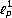Theory and Modern Applications

# A functional-analytic method for the study of difference equations

## Abstract

We will give the generalization of a recently developed functional-analytic method for studying linear and nonlinear, ordinary and partial, difference equations in theandspaces, p,. The method will be illustrated by use of two examples concerning a nonlinear ordinary difference equation known as the Putnam equation, and a linear partial difference equation of three variables describing the discrete Newton law of cooling in three dimensions.

## Author information

Authors

### Corresponding author

Correspondence to Eugenia N Petropoulou.

## Rights and permissions

Reprints and Permissions

Petropoulou, E.N., Siafarikas, P.D. A functional-analytic method for the study of difference equations. Adv Differ Equ 2004, 537067 (2004). https://doi.org/10.1155/S1687183904310101